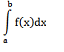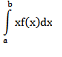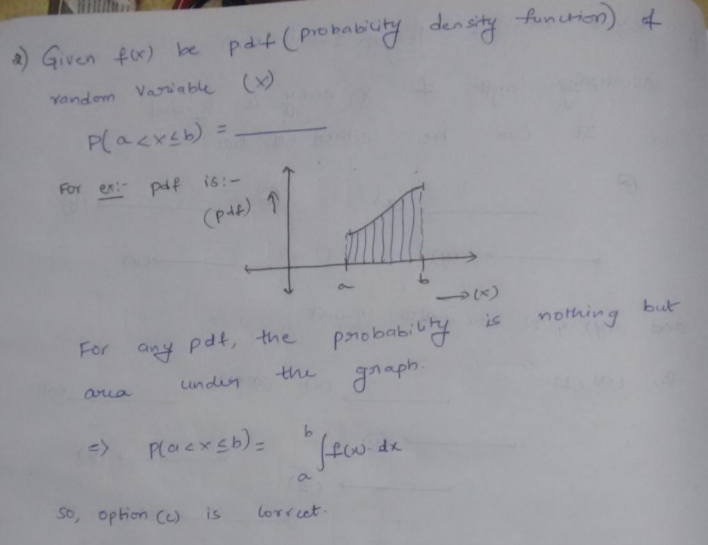# GATE | GATE-CS-2005 | Question 12

• Last Updated : 28 Jun, 2021

Let f(x) be the continuous probability density func­tion of a random variable X. The probability that a < X ≤ b, is

```
A) f(b - a)
B) f(b) - f(a)
C)D)```

(A) A
(B) B
(C) C
(D) D

Explanation:This solution is contributed by Anil Saikrishna Devarasetty.

Quiz of this Question

My Personal Notes arrow_drop_up